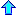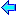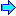# Current projects

### Magnetohydrodynamics

For several years I have been working (with P. G. Schmidt) on Magnetohydrodynamics (MHD). This is the theory of the macroscopic interaction of electrically conducting fluids with a magnetic field. It is of importance in connection with many engineering problems (in particular metallurgy) such as plasma confinement, liquid-metal cooling of nuclear reactors, electromagnetic casting and aluminum production. It also finds applications in geophysics and astronomy.

This work is partially funded by grants from the NSF.

We have devised a new formulation of the MHD equations (a coupled system of nonlinear partial differential equations) and were able to answer some theoretical questions pertaining to the equivalence of this new formulation to the standard one and its well-posedness (we also proved existence and uniqueness results and continuous dependence on data). The main reason for considering and devising this new formulation are its advantages for numerical simulations of MHD flows.

I have implemented a finite element (computer) program (which uses this new formulation) that approximates solutions of the MHD equations. In collaboration with Paul Gray (Emory University) we are working on a parallelizable version of the code. This code will be used on supercomputer-class computers to simulate phenomena of interest to scientists and engineers.

### CFD The Stokes and Navier Stokes Equations

Recently I have been working on developing better differencing schemes novel formulations and more efficient iterative solvers for approximating the solutions of the Navier Stokes equations (a coupled system of nonlinear partial differential equations describing the flow of viscous incompressible fluid).

I have developed an efficient finite element algorithm for approximating solutions of the Stokes equation. This algorithm will be extended in the near future to the nonlinear Navier Stokes equations.

### Geophysical Flows

In collaboration with Irad Yavneh (Technion - Israel Institute of Technology) I have been studying the three-dimensional Quasigeostrophic equations, which model large-scale rotating stratified flows. We are interested in developing efficient algorithms for the numerical simulation of geophysical flows which are governed by these equations.

### HyperTeX

I am very much interested in HyperTeX, a combination of an hypertext markup language and the mathematical typesetting system TeX. I think HyperTeX will have a major impact on (electronic) publication of mathematical an scientific works. It should also be an interesting educational tool.HomeSelected PublicationsTeaching Philosophyajm@math.auburn.edu